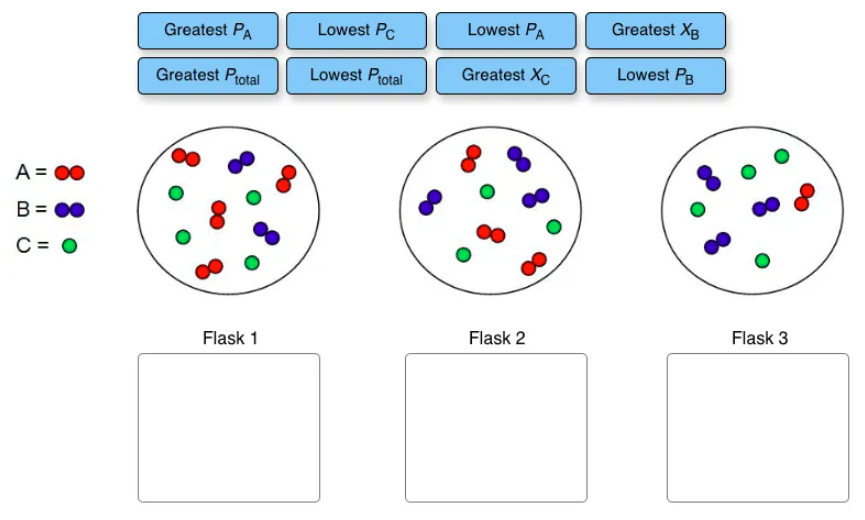# Problem: Assign each of the given labels to the flask that it correctly describes.

###### FREE Expert Solution

We’re being asked to assign each of the given labels to the flask that it correctly describes.

Recall that the partial pressure of a gas (Pgas) in a mixture is given by:

$\overline{){\mathbf{P}}_{\mathbf{gas}}\mathbf{=}{\mathbf{\chi }}_{\mathbf{gas}}{\mathbf{P}}_{\mathbf{total}}}$

χgas = mole fraction of the gas
Ptotal = total pressure of the gas mixture.

The mole fraction of the gas is then given by:

89% (444 ratings)###### Problem Details

Assign each of the given labels to the flask that it correctly describes.